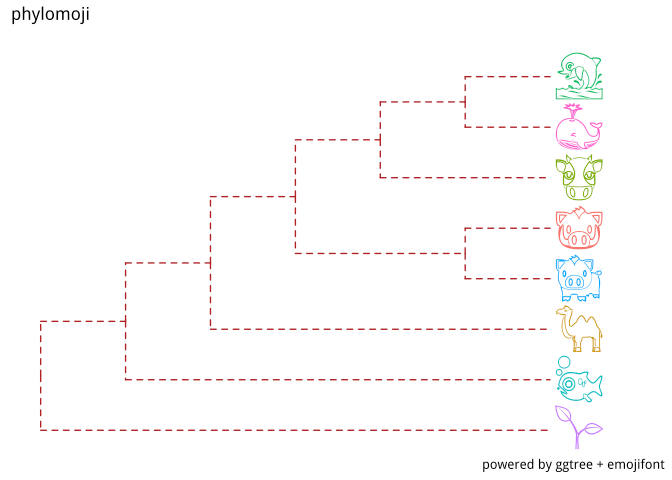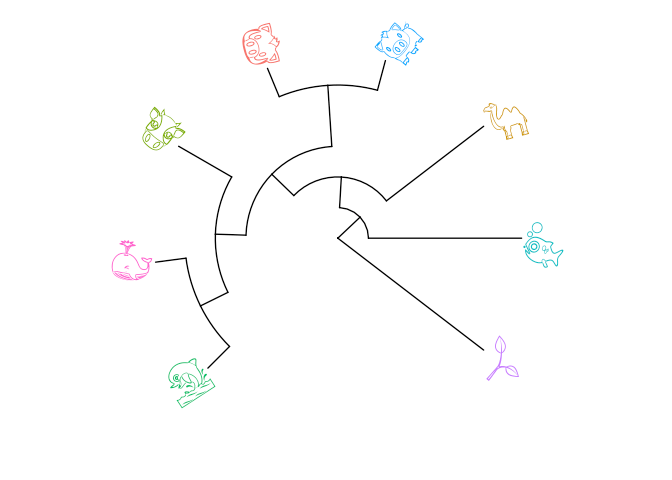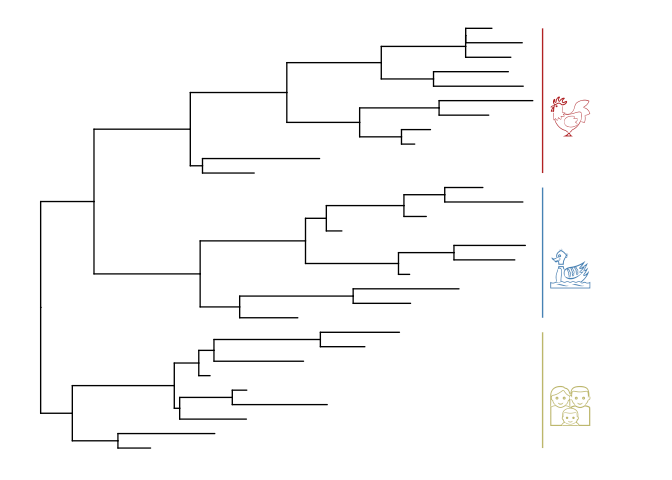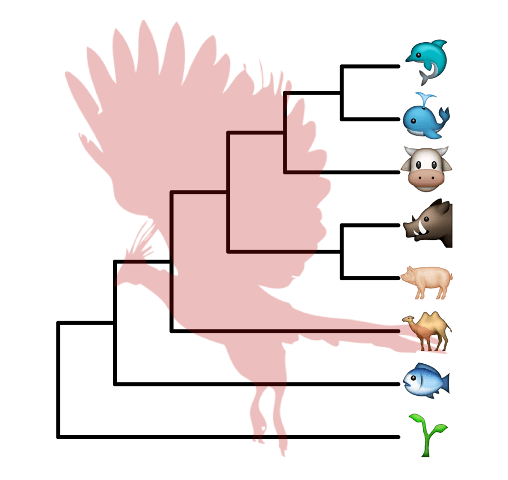Want to share your content on R-bloggers? click here if you have a blog, or here if you don't.

With ggtree (Yu et al. 2017), it is very easy to create phylomoji. Emoji is internally supported by ggtree.

## Use emoji in taxa labels

``````library(ggtree)
tree_text <- "(((((cow, (whale, dolphin)), (pig2, boar)), camel), fish), seedling);"
ggtree(x, linetype="dashed", color='firebrick') +
xlim(NA, 7) + ylim(NA, 8.5) +
geom_tiplab(aes(color=label), parse='emoji', size=14, vjust=0.25) +
````````````p <- ggtree(x, layout='circular') +
geom_tiplab2(aes(color=label), parse='emoji', size=12, vjust=0.25)
print(p)
````````````open_tree(p, angle=200)
````````````open_tree(p, angle=60) %>% rotate_tree(-75)
````````````set.seed(123)
tr <- rtree(30)
ggtree(tr) + xlim(NA, 5) +
fontsize=12, align=TRUE, color="firebrick") +
fontsize=12, align=TRUE, color="steelblue") +
fontsize=12, align=TRUE, color="darkkhaki")
``````## Apple Color Emoji

Although `R`’s graphical devices don’t support `AppleColorEmoji` font, it’s still possible to use it. We can export the `plot` to `svg` file and render it in `Safari`.

``````library(ggimage)
library(gridSVG)
p <-  ggtree(x, size=2) + geom_tiplab(size=20, parse='emoji') +
xlim(NA, 7) + ylim(NA, 8.5) +
color="firebrick", alpha = .3, size=Inf)

p
``````Please visit ggtree homepage, https://guangchuangyu.github.io/ggtree, to find out more.

# References

Yu, Guangchuang, David Smith, Huachen Zhu, Yi Guan, and Tommy Tsan-Yuk Lam. 2017. “Ggtree: An R Package for Visualization and Annotation of Phylogenetic Trees with Their Covariates and Other Associated Data.” Methods in Ecology and Evolution 8 (1): 28–36. doi:10.11112041-210X.12628.